# Сборник текстов на казахском, русском, английском языках для формирования навыков по видам речевой деятельности обучающихся уровней среднего образования

 бет 40/65 Дата 05.11.2016 өлшемі 17,36 Mb.

## Reaction Rate

Chemical reactions vary greatly in the speed at which they occur. Some are essentially instantaneous, while others may take years to reach equilibrium. The Reaction Rate for a given chemical reaction is the measure of the change in concentration of the reactants or the change in concentration of the products per unit time.

#### Definition of reaction rate

The speed of a chemical reaction may be defined as the change in concentration of a substance divided by the time interval during which this change is observed: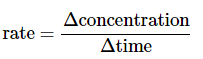For a reaction of the form A+B→CA+B→C, the rate can be expressed in terms of the change in concentration of any of its components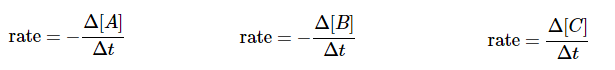in which Δ[A] is the difference between the concentration of A over the time interval t2 – t1: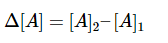Notice the minus signs in the first two examples above. The concentration of a reactant always decreases with time, so Δ[A]and Δ[A] are both negative. Since negative rates do not make much sense, rates expressed in terms of a reactant concentration are always preceded by a minus sign to make the rate come out positive.

Consider now a reaction in which the coefficients are different:

A+3B→2D

It is clear that [B] decreases three times as rapidly as [A], so in order to avoid ambiguity when expressing the rate in terms of different components, it is customary to divide each change in concentration by the appropriate coefficient: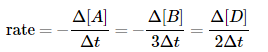Example 1

For the oxidation of ammonia

4NH3+3O2→2N2+6H2O

it was found that the rate of formation of N2 was 0.27 mol L–1 s–1.

1. At what rate was water being formed?

2. At what rate was ammonia being consumed?

SOLUTION

a) From the equation stoichiometry, Δ[H2O] = 6/2 Δ[N2], so the rate of formation of H2O is

3 × (0.27 mol L–1 s–1) = 0.81 mol L–1 s–1.

b) 4 moles of NH3 are consumed for every 2 moles of N2 formed, so the rate of disappearance of ammonia is

2 × (0.27 mol L–1 s–1) = 0.54 mol L–1 s–1.

Comment: Because of the way this question is formulated, it would be acceptable to express this last value as a negative number.

#### Instantaneous rates

Most reactions slow down as the reactants are consumed. Consequently, the rates given by the expressions shown above tend to lose their meaning when measured over longer time intervals Δt. Note: Instantaneous rates are also known as differential rates.

Thus for the reaction whose progress is plotted here, the actual rate (as measured by the increasing concentration of product) varies continuously, being greatest at time zero. The instantaneous rate of a reaction is given by the slope of a tangent to the concentration-vs.-time curve.

An instantaneous rate taken near the beginning of the reaction (t = 0) is known as an initial rate (label (1)here). As we shall soon see, initial rates play an important role in the study of reaction kinetics. If you have studied differential calculus, you will know that these tangent slopes are derivatives whose values can very at each point on the curve, so that these instantaneous rates are really limiting rates defined as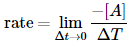If you do not know calculus, bear in mind that the larger the time interval Δt, the smallerwill be the precision of the instantaneous rate.

### Introduction

During the course of the reaction shown below, reactants A and B are consumed while the concentration of product AB increases.  The reaction rate can be determined by measuring how fast the concentration of A or B decreases, or by how fast the concentration of AB increases.

A+B⟶AB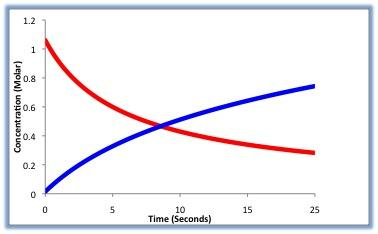Figure 1.  The above picture shows a hypthetical reaction profile in which the reactants (red) decrease in concentration as the products increase in concetration (blue).

For the stochiometrically complicated Reaction: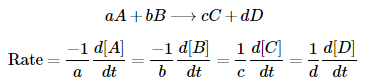Looking at Figure 1 above, we can see that the rate can be measured in terms of either reactant (A or B) or either product (C or D). Not all variables are needed to solve for the rate. Therefore, if you have the value for "A" as well as the value for "a" you can solve for the reaction rate.

You can also notice from Formula 1 above that, the change in reactants over the change in time must have a negative sign in front of them. The reason for this is because the reactants are decreasing as a function of time, the rate would come out to be negative (because it is the reverse rate). Therefore, putting a negative sign in front of the variable will allow for the solution to be a positive rate.

### Rate Laws and Rate Constants

A rate law is an expression which relates that rate of a reaction to the rate constant and the concentrations of the reactants.  A rate constant, kk, is a proportionality constant for a given reaction.  The general rate law is usually expressed as:

Rate=k[A]s[B]t

As you can see from equation 2 above, the reaction rate is dependent on the concentration of the reactants as well as the rate constant. However, there are also other factors that can influence the rate of reaction. These factors include temperature and catalysts. When you are able to write a rate law equation for a certain reaction, you can determine the Reaction Order based on the values of s and t.

### Temperature Dependence

#### Reaction Rate

If you were to observe a chemical reaction to occur in two different setting (one at a higher temperature than the other), you would most likely observe the reaction occuring at a higher temperature to have a higher rate. This is because as you increase the temperature, the kinetic energy of the reactants increase, allowing for more collisions between the molecules. This, therefore, allows for products to be formed faster. A simple rule of thumb that can be used is: for every 10°C increase, the reaction rate doubles.

However, increasing the temperature will not always increase the rate of the reaction. If the temperature of a reaction were to reach a certain point where the reactant will begin to degrade, it will decrease the rate of the reaction.

#### Rate Constant

As stated in the note above, the rate constant, k, is dependent on the temperature of which the reaction takes place. This can be seen through the Arrhenius Equation shown below:

k=AeEa/RT

As you can see from equation 3, the rate constant kk is dependent on the temperature (in Kelvins) and also the Activation energy, Ea(in joules). "A" in the equation represents a pre-exponential factor that has the same units as k. Finally, R is the universal gas constant.

### Catalysts

Catalysts are a class of molecules which lower the activation energy (Ea) required for reactants to collide and form products. They are not consumed in the reaction themselves, therefore they are only there to basically assist the reaction is progressing forward. Thus, catalysts increase the rate of reaction. The most common type of catalyst is the Enzyme Catalyst. In chemical reactions, products are formed when reactants collide with one another. Enzymes allow for reactants to collide in perfect orienation making the reaction more effective in forming products.

### Reaction Order

The reaction rate for a given reaction is a crucial tool that enables us to calculate the specific order of a reaction. The order of a reaction is important in that it enables us to classify specific chemical reactions easily and efficiently. Knowledge of the reaction order quickly allows us to understand numerous factors within the reaction including the rate law, units of the rate constant, half life, and much more. Reaction order can be calculated from the rate law by adding the exponential values of the reactants in the rate law. It is important to note that although the reaction order can be determined from the rate law, there is no relationship between the reaction order and the stoichiometric coefficients in the chemical equation.

Example 1:

Rate=k[A]s[B]t

Reaction Order=s+t

NOTE: The rate of reaction must be a non-negative value. It can be zero and does not need to be an integer.

As shown in Formula 5, the complete reaction order is equal to the sum of "s" and "t." But what does each of these variables by themself mean? Each variable represents the order of the reaction with respect to the reactant it is placed on. In this certain situation, s is the order of the reaction with respect to [A] and t is the order of the reaction with respect to [B].

Here is an example of how you can look at this: If a reaction order with respect to [A] was 2 (s = 2) and [B] was 1 (t = 1), then that basically means that the concentration of reactant A is decreasing by a factor of 2 and the concentration of [B] is decrease by a factor of 1.

So if you have a reation order of Zero (s + t = 0), this basically means that the concentration of the reactants does not affect the rate of reaction. You could remove or add reactants to the mixture but the rate will not change.

A list of the different reaction rate equations for zero-, first-, and second-order reactions can be seen in Table 1. This table also includes further equations that can be determine by this equation once the order of the reaction is known (Half life, integrated rate law, etc.)
 Table 1. The table below displays numerous values and equations utilized when observing chemical kinetics for numerous reactions types Zero-Order First-Order Second-Order Rate Law Rate= k Rate= k[A] Rate= k[A]2 Integrated Rate Law [A]t= −kt+[A]0 ln[A]t= −kt+ln[A]0 1[A]t=−kt+1[A]0 Units of Rate Constant (k): molL−1s−1 s−1 Lmol−1s−1 Linear Plot to Determine (k): [A] versus time ln[A] versus time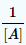versus time Relationship of Rate Constant to the Slope of Straight Line: slope= −k slope= −k slope= k Half-life: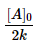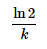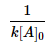### Sample Problems

1. Define Reaction Rate

2. TRUE or FALSE: Changes in the temperature or the introduction of a catalyst will affect the rate constant of a reaction

For sample problems 3-6, use Formula 6 to answer the questions

H2O⟶2H2+O2(6)(6)H2O⟶2H2+O2

*Assume the reaction occurs at constant temperature

3. For the given reaction above, state the rate law.

4. State the overall order of the reaction.

5. Find the rate, given k = 1.14 x 10-2 and [H2O] = 2.04M

6. Find the half-life of the reaction.

1. Reaction Rate is the measure of the change in concentration of the disappearance of reactants or the change in concentration of the appearance of products per unit time.

2. FALSE. The rate constant is not dependant on the presence of a catalyst. Catalysts, however, can effect the total rate of a reaction.

3.  Rate= k[H2O] Rate= k[H2O]

4. First - Order

5. 2.33 x 10-2 s-1

6. 29.7 s

3.3. A chemical equation is the symbolic representation of a chemical reaction in the form of symbols and formulae, wherein the reactant entities are given on the left-hand side and the product entities on the right-hand side. The coefficients next to the symbols and formulae of entities are the absolute values of the stoichiometric numbers. The first chemical equation was diagrammed by Jean Beguin in 1615.

A chemical equation consists of the chemical formulas of the reactants (the starting substances) and the chemical formula of the products (substances formed in the chemical reaction). The two are separated by an arrow symbol ({\displaystyle \rightarrow }, usually read as "yields") and each individual substance's chemical formula is separated from others by a plus sign.

As an example, the equation for the reaction of hydrochloric acid with sodium can be denoted:

2 HCl +2 Na →2 NaCl + H 2

This equation would be read as "two HCl plus two Na yields two NaCl and H two." But, for equations involving complex chemicals, rather than reading the letter and its subscript, the chemical formulas are read using IUPAC nomenclature. Using IUPAC nomenclature, this equation would be read as "hydrochloric acid plus sodium yields sodium chloride andhydrogen gas."

This equation indicates that sodium and HCl react to form NaCl and H2. It also indicates that two sodium molecules are required for every two hydrochloric acid molecules and the reaction will form two sodium chloride molecules and one diatomic molecule of hydrogen gas molecule for every two hydrochloric acid and two sodium molecules that react. Thestoichiometric coefficients (the numbers in front of the chemical formulas) result from the law of conservation of mass and the law of conservation of charge

Chemical reactions happen all around us: when we light aMATCH, start a car, eat dinner, or walk the dog. A chemical reaction is the process by which substances bond together (or break bonds) and, in doing so, either release or consume energy (see our Chemical Reactions module). A chemical equation is shorthand that scientists use to describe a chemical reaction. Let's take the reaction of hydrogen with oxygen to form water as an example. If we had a container of hydrogen gas and burned this in the presence of oxygen, the two gases would react together, releasing energy, to form water. To write the chemical equation for this reaction, we would place the substances reacting (the reactants) on the left side of an equation with an arrow pointing to the substances being formed on the right side of the equation (the products). Given this information, one might guess that the equation for this reaction is written:
 H + O → H2O

The plus sign on the left side of the equation means that hydrogen (H) and oxygen (O) are reacting. Unfortunately, there are two problems with this chemical equation. First, because atoms like to have full valence shells, single H or O atoms are rare. In nature, both hydrogen and oxygen are found asdiatomic molecules, H2 and O2, respectively (in forming diatomic molecules the atoms shareelectrons and complete their valence shells). Hydrogen gas, therefore, consists of H2 molecules; oxygen gas consists of O2. Correcting our equation we get:
 H2 + O2 → H2O

But we still have one problem. As written, this equation tells us that one hydrogen molecule (with two H atoms) reacts with one oxygen molecule (two O atoms) to form one water molecule (with two Hatoms and one O atom). In other words, we seem to have lost one O atom along the way! To write a chemical equation correctly, the number of atoms on the left side of a chemical equation has to be precisely balanced with the atoms on the right side of the equation. How does this happen? In actuality, the O atom that we "lost" reacts with a second molecule of hydrogen to form a second molecule of water. During the reaction, the H-H and O-O bonds break and H-O bonds form in the water molecules, as seen in the simulation below.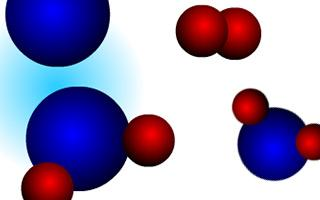Interactive Animation:The formation of water
The balanced equation is therefore written:
 2H2 + O2 → 2H2O

In writing chemical equations, the number in front of the molecule's symbol (called a coefficient) indicates the number of molecules participating in the reaction. If no coefficient appears in front of a molecule, we interpret this as meaning one.

In order to write a correct chemical equation, we must balance all of the atoms on the left side of thereaction with the atoms on the right side. Let's look at another example. If you use a gas stove to cook your dinner, chances are that your stove burns natural gas, which is primarily methane. Methane (CH4) is a molecule that contains four hydrogen atoms bonded to one carbon atom. When you lightthe stove, you are supplying the activation energy to start the reaction of methane with oxygen in the air. During this reaction, chemical bonds break and re-form and the products that are produced are carbon dioxide and water vapor (and, of course, light and heat that you see as the flame). The unbalanced chemical equation would be written:

 CH4 (methane) + O2 (oxygen) → CO2 (carbon dioxide) + H2O (water)

Look at the reaction atom by atom. On the left side of the equation we find one carbon atom, and one on the right.
 C H4 + O2 → C O2 + H2 O ↑ 1 carbon ↑ 1 carbon

Next we move to hydrogen: There are four hydrogen atoms on the left side of the equation, but only two on the right.
 C 4 + 2 → C O2 + H2 O ↑ 4 hydrogen ↑ 2 hydrogen

Therefore, we must balance the H atoms by adding the coefficient "2" in front of the water molecule(you can only change coefficients in a chemical equation, not subscripts). Adding this coefficient we get:
 C H4 + O2 → C O2 + 2H2 O ↑ 4 hydrogen ↑ 4 hydrogen

What this equation now says is that two molecules of water are produced for every one molecule of methane consumed.  Moving on to the oxygen atoms, we find two on the left side of the equation, but a total of four on the right side (two from the CO2 molecule and one from each of two water molecules H2O).
 C H4 + O2 → C O2 + 2H2 O ↑ 2 oxygen ↑ 4 oxygen

To balance the chemical equation we must add the coefficient "2" in front of the oxygen molecule on the left side of the equation, showing that two oxygen molecules are consumed for every one methane molecule that burns.
 C H4 + 2O2 → C O2 + 2H2 O ↑ 4 oxygen ↑ 4 oxygen

Dalton's law of definite proportions holds true for all chemical reactions (see our Early Ideas about Matter: From Democritus to Dalton module). In essence, this law states that a chemical reactionalways proceeds according to the ratio defined by the balanced chemical equation. Thus, you can interpret the balanced methane equation above as reading, "one part methane reacts with two parts oxygen to produce one part carbon dioxide and two parts water." This ratio always remains the same. For example, if we start with two parts methane, then we will consume four parts O2 and generate two parts CO2 and four parts H2O. If we start with excess of any of the reactants (e.g., five parts oxygen when only one part methane is available), the excess reactant will not be consumed:
 C H4 + 5O2 → C O2 + 2H2 + 0 + 3O2 Excessreactantswill not be consumed.

In the example seen above, 3O2 had to be added to the right side of the equation to balance it and show that the excess oxygen is not consumed during the reaction. In this example, methane is called the limiting reactant.

Although we have discussed balancing equations in terms of numbers of atoms and molecules, keep in mind that we never talk about a single atom (or molecule) when we use chemical equations. This is because single atoms (and molecules) are so tiny that they are difficult to isolate. Chemical equations are discussed in relation to the number of moles of reactants and products used or produced (see our The Mole module). Because the mole refers to a standard number of atoms (or molecules), the term can simply be substituted into chemical equations. Thus, the balanced methane equation above can also be interpreted as reading, "one mole of methane reacts with two moles of oxygen to produce one mole of carbon dioxide and two moles of water."

### Lewis Theory

The Lewis definition is the most general theory, having no requirements for solubility or protons.

Lewis Acids and Bases

1. An acid is a substance that accepts a lone pair of electrons.

2. A base is a substance that donates a lone pair electrons.

Lewis acids and bases react to create an adduct, a compound in which the acid and base have bonded by sharing the electron pair. Lewis acid/base reactions are different from redox reactions because there is no change in oxidation state.This reaction shows a Lewis base (NH3) donating an electron pair to a Lewis acid (H+) to form an adduct (NH4+).

Достарыңызбен бөлісу: